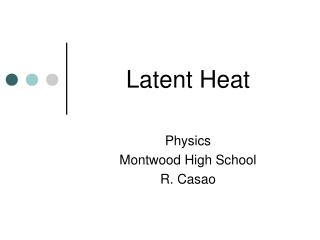DownloadDownload PresentationLatent Heat

# Latent Heat

Télécharger la présentation## Latent Heat

- - - - - - - - - - - - - - - - - - - - - - - - - - - E N D - - - - - - - - - - - - - - - - - - - - - - - - - - -
##### Presentation Transcript

1. Latent Heat Physics Montwood High School R. Casao

2. Change of State • Matter exists in 3 states: • Solid • Liquid • Gas Consider water: vaporization(takes place at boiling point) fusion(at melting point) ice water steam solidification condensation at freezing point at boiling point

3. Heats of Transformation • When energy is absorbed as heat by a solid or liquid, the temperature of the object does not necessarily rise. • The thermal energy may cause the mass to change from one phase, or state, to another. • The amount of energy per unit mass that must be transferred as heat when a mass undergoes a phase change is called the heat of transformation, L.

4. Heat of Fusion • To melt a solid means to change its from the solid state to the liquid state. This process requires energy because the molecules of the solid must be freed from their rigid structure. • To freeze a liquid to form a solid is the reverse of melting and requires that energy be removed from the liquid so that the molecules can settle into a rigid structure.

5. Heat of Fusion • When the phase change is from solid to liquid, the sample must absorb heat; when the phase change is from a liquid to solid, the sample must release heat. • The heat of transformation for these phase changes is called the heat of fusion, Lf. • Water: Lf = 334 J/g = 79.5 cal/g

6. Heat of Vaporization • To vaporize a liquid means to change it from the liquid state to the vapor or gas state. This process requires energy because the molecules must be freed from the liquid state. • Condensing a gas to a liquid is the reverse of vaporizing; it requires that energy be removed from the gas so that the molecules can cluster together instead of flying away from each other. • The heat of transformation for these phase changes is called the heat of vaporization, Lv. • Water: Lv = 2256 J/g = 539 cal/g

7. Phase Changes

8. Gas-Liquid Equilibration Chapter 11

9. Phase Changes Energy Changes Accompanying Phase Changes Chapter 11

10. Phase Changes Heating Curves Chapter 11

11. Heating Curve Illustrated Chapter 11

12. Phase Change Diagram - Water

13. condensation energy out 2260 kJ energy out 334 kJ solidification Summary: Change of State vaporization energy in 2260 kJ steam (1 kg) water (1 kg) energy in 334 kJ ice (1 kg) fusion

14. ice (ice and water) melting boiling (water and stream) (334) (2260) water Summary: from ice to steam steam 100 0 energy / kJ (420) Energy involved in heating 1 kg of water

15. Heat of Combustion (HC) • The amount of heat released per unit mass or unit volume of a substance when the substance is completely burned. • Heats of combustion are used as a basis for comparing the heating value of fuels, since the fuel that produces the greater amount of heat for a given cost is the more economic. • Equation:

16. Phase Change Example • Heat is added to 0.5 kg of water at 20° C. How many joules of heat energy are required to change the water to steam at 110° C? • Heat has to be added to the water to raise its temperature from 20° C to the boiling point 100° C. • Heat has to be added to vaporize all of the water that is at 100° C.

17. Phase Change Example • Heat has to be added to raise the temperature of all of the steam from 100° C to 110° C. • Total heat energy: Q = 167440 J + 1130000 J + 10050 J = 1307490 J

18. Final Temperature Example • A 0.3 kg piece of ice at 0° C is placed in 1 kg of water at 40° C in an insulated container. If no heat is lost to the container, what is the final temperature of the water? • Q lost water = Q gained ice + Q gained ice water • (m·c·DT)water = m·Lf + (m·c·DT)ice water

19. Final Temperature Example